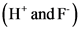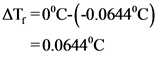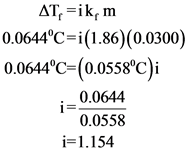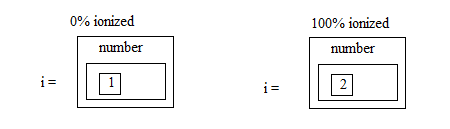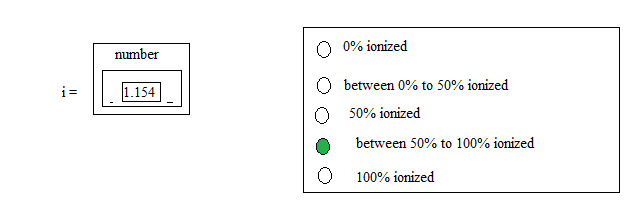# For an aqueous solution of hf. determine the van’t hoff factor assuming…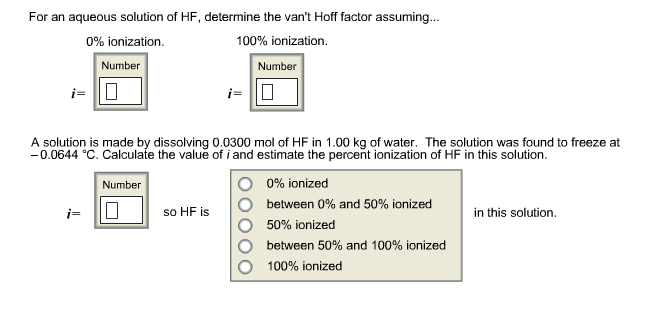For an aqueous solution of HF, determine the van’t Hoff factor assuming… A solution is made by dissolving 0.0300 mol of HF in 1.00 kg of water. The solution was found to freeze at -0.0644 degree C. Calculate the value of i and estimate the percent ionization of HF in this solution.

General guidance

Concepts and reason
Colligative properties: Colligative properties are properties that depend on the ratio of solute particles to solvent particles in a mixed volatile and non-volatile solution. These properties do not depend on the nature of the solute particles. Colligative properties are given below.
1.Relative lowering of vapor pressure
2.Boiling point elevation
3.Freezing point depression
4.Osmotic pressure

Fundamentals

• Elevation of boiling point: Boiling point for a pure substance is increased by adding non-volatile solute to it.

• Freezing point depression: Freezing point for a pure substance is lowered by adding insoluble substances to it.

Van’t Hoff factor for the substance is the number of ions present in that solution.

Step-by-step

Step 1 of 2

Given solution is aqueous solution of HF.
If the solution is not ionized (0% ionization), then the van’t Hoff factor value is 1 since only HF molecules are present in the solution.
If the solution is fully ionized (100% ionization), then the van’t Hoff factor value is 2 since there are two ions that are present in the solution.
Therefore, ‘i’ value is 1 for 0% ionization and ‘i’ value is 2 for complete ionization.

Van’t Hoff factor values have been found for completely ionized condition and non-ionized condition of aqueous solution of HF. Non-ionized aqueous solution of HF has only HF molecules in the solution, so the van’t Hoff factor value is 1. Completely ionized solution has two ions in the solution, so the van’t Hoff factor is 2.

Take care while giving the van’t Hoff factor for different solutions.
Example:
Correct:
Van’t Hoff factor is 1 for non-ionized aqueous solution.
Van’t Hoff factor is 2 for completely ionized aqueous solution.
Incorrect:
Van’t Hoff factor is 2 for non-ionized aqueous solution.
Van’t Hoff factor is 1 for completely ionized aqueous solution.

Find the van’t Hoff factor of the aqueous solution of HF where 0.0300 mole in 1kg water.

Step 2 of 2

Given reaction condition is written below.
A solution is prepared by dissolving 0.0300 mole of HF in 1.00kg of water.
Cryoscopic constant for water is 1.86
Freezing point of the solution is -0.06440C
Depression in freezing point value is shown below.

Molality of the solution is calculated below.

Van’t Hoff factor for the solution is calculated below.

Therefore, van’t Hoff factor for the given solution is 1.154
It is clear from the van’t Hoff factor that the solution is ionized more than 50%.
[Part B]

Part B
Van’t Hoff factor for an aqueous solution of HF

Van’t Hoff factor of the solution, which is prepared by addition of 0.300 moles of HF in 1kg of water

Van’t Hoff factor has been found for the given solution by using the colligative property depression in freezing point. Van’t Hoff factor is more than 1, which means that the solution is more than 50% ionized since the complete ionization is 2.

Take care while giving the van’t Hoff factor value for the prepared solution.
Example:
Correct:
Van’t Hoff factor is 1.154 for prepared solution.
Incorrect:
Van’t Hoff factor is 2 for prepared solution.

Part B
Van’t Hoff factor for an aqueous solution of HF

Van’t Hoff factor of the solution, which is prepared by addition of 0.300 moles of HF in 1kg of water

Part B
Van’t Hoff factor for an aqueous solution of HF

Van’t Hoff factor of the solution, which is prepared by addition of 0.300 moles of HF in 1kg of water

AT,=ik, m AT, =elevation of boiling point k=cryoscopic constant i= van’t Hoff factor m=molality
AT, Eik, m AT, = freezing point depression k, =cryoscopic constant i=van’t Hoff factor m=molality
(H and F)
(H and F)
AT, =0°C-(-0.0644°C) =0.0644°C
number of moles molality= mass of the solvent 0.0300 1Kgof water =0.0300m
AT, =ik, m 0.0644°C=i(1.86)(0.0300) 0.0644°C=(0.0558°C)i i = 0.0644 0.0558 i=1.154
0% ionized 100% ionized number number
0 0% ionized number 1.154 O o between 0% to 50% ionized 50% ionized between 50% to 100% ionized 100% ionized O
0% ionized 100% ionized number number
0 0% ionized number 1.154 O o between 0% to 50% ionized 50% ionized between 50% to 100% ionized 100% ionized O